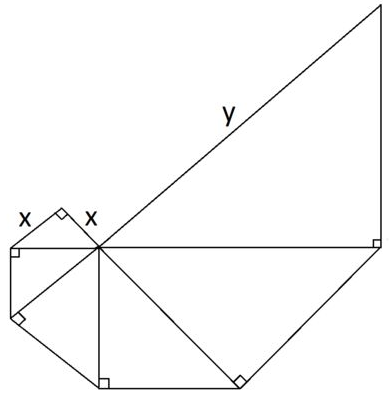# Spiraling Triangles

Geometry Level 2In the diagram above, each triangle is a right isosceles triangle. What is the value of $y$ (in terms of $x$)?

Note: An isosceles triangle is a triangle in which two of the sides have the same length.

Bonus: What would the value of the largest side be if the spiraling pattern of right isosceles triangles established in the diagram was continued to include 14 triangles?

×